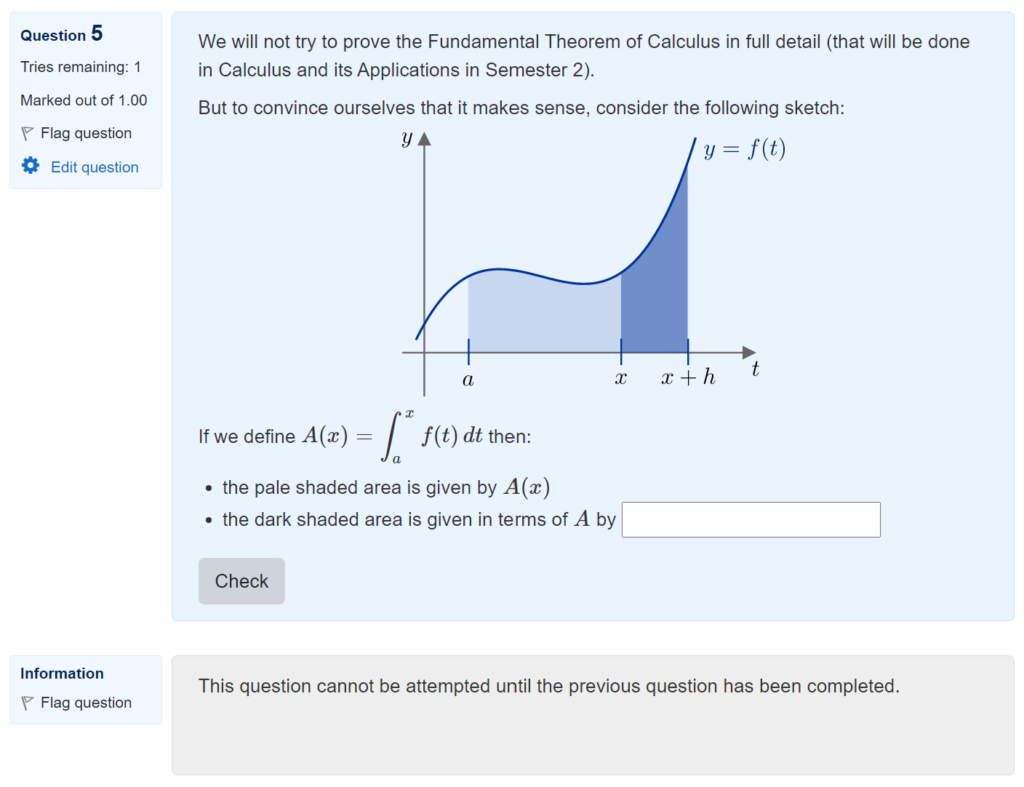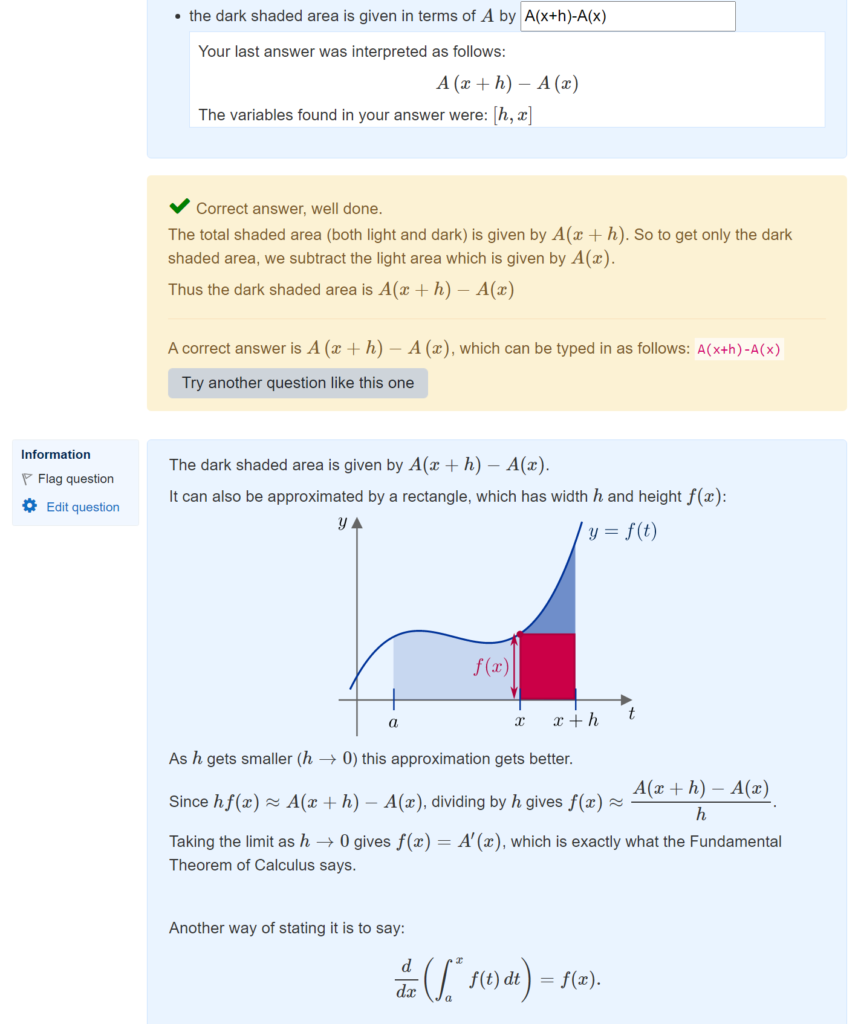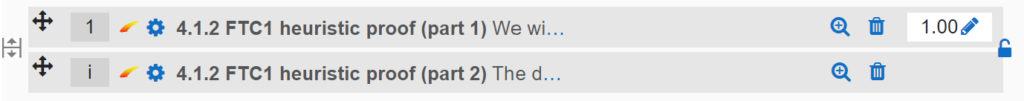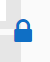## Scaffolded proofs in a Moodle quiz

In my online course Fundamentals of Algebra and Calculus, there were several places where I wanted to encourage students to engage with a key proof while reading the text.

One approach to this is to ask proof comprehension questions after giving the proof, but I’ve also tried writing some sequences of questions that lead the students through the proof in a scaffolded/structured way.

Here’s a simple example, of a sketch proof of the Fundamental Theorem of Calculus:Students can’t see the next part of the proof until they give an answer. Once they have submitted their answer, the next part is revealed:I’ve used this approach in other places in the course, sometimes with more than one step.

The way to do this in Moodle is by having the quiz settings set to “Interactive with multiple tries”:Then using the little padlock symbols that appear at the right-hand side between questions on the “Edit questions” page:After clicking the padlock, it changes to locked to indicate that students must answer the first question to see the second:I’ve not done any serious evaluation of this approach, but my intuition is that it’s a good way to direct students’ attention to certain parts of a proof and encourage them to be more active in their reading.

/

## STACK: Checking answers in polar form

Last week’s topic in FAC was complex numbers, and I’ve had some difficulties with STACK questions asking students to give their answer in polar form, e.g. when the correct answer was `4*(cos(pi/3)+i*sin(pi/3))` an answer of `4*(cos((1/3)*pi)+i*sin((1/3)*pi))` would be marked incorrect!

The issue was that:

• with simplification turned on, Maxima will automatically simplify polar form to cartesian form, so I need simplification off.
• with simplification off, Maxima won’t see those equally valid ways of writing the argument as the same.

I was using the EqualComAss answer test to check whether the student answer (ans1) was equal to the model answer (ta1), and this was failing in the cases above.

The solution I came up with is to add some code to the feedback variables box at the top of the PRT, to replace cos and sin with alternate versions so that Maxima can’t simplify the expressions to cartesian form. I can then use ev(…,simp) to make use of simplification when comparing the expressions:

```form_ans1:subst([cos=COSINE, sin=SINE], ans1);
form_ta1:subst([cos=COSINE, sin=SINE], ta1);
proper_form:is(ev(expand(form_ans1-form_ta1),simp)=0);```

This will ensure that COSINE(pi/3) and COSINE((1/3)*pi) will cancel out, thanks to the simplification being turned on.

But since Maxima doesn’t know anything about COSINE, it can’t cancel out COSINE(-pi/3) and COSINE(5pi/3) (as it would do with cos) if students give their answer with the wrong value for the principal argument.

It was then just a case of replacing the test for EqualComAss(ans1,ta1) in the PRT with a test that AlgEquiv(proper_form, true), and regrading. Out of ~160 attempts this picked up 8 students who deserved full marks!

Update (08/11/2021): One year on, and STACK now has a new feature which makes it easier to grade these answers correctly! The new EqualComAssRules answer test lets you add a list of different algebraic rules so that two answers should count as equivalent if they differ only by those rules – e.g. x and 1*x.

To fix this question, it’s enough to change the first PRT node to the following, using the “Test options” box to specify the list of algebraic rules:

`ATEqualComAssRules(ans1, ta1, [ID_TRANS,NEG_TRANS,DIV_TRANS,INT_ARITH]);`
/